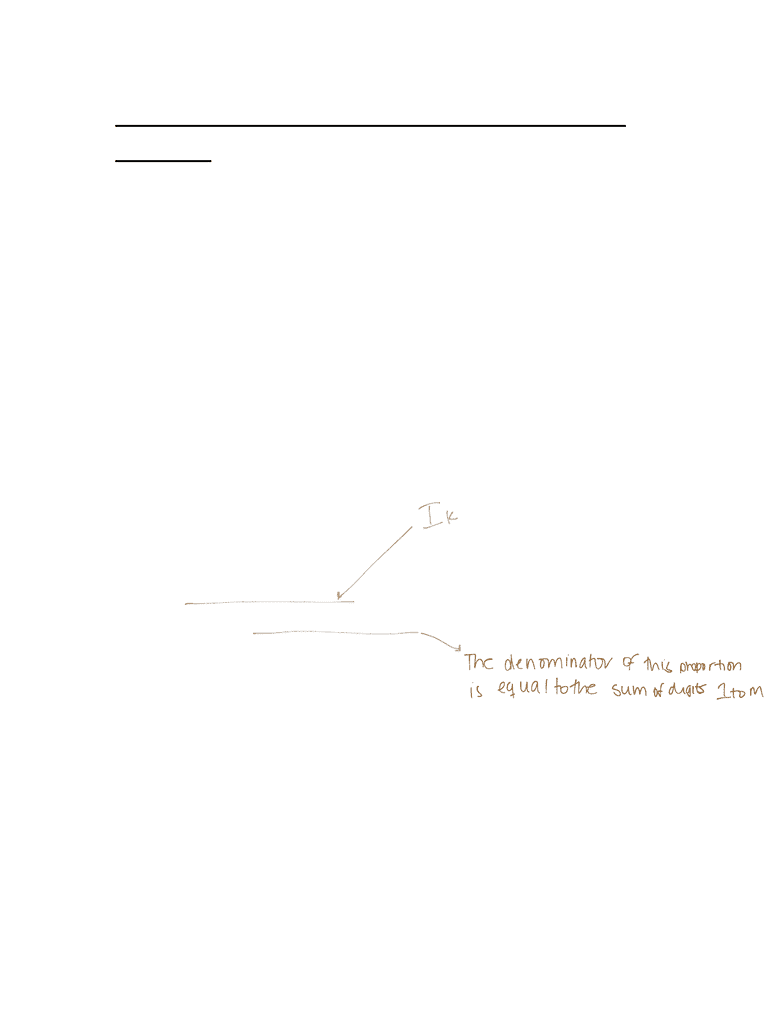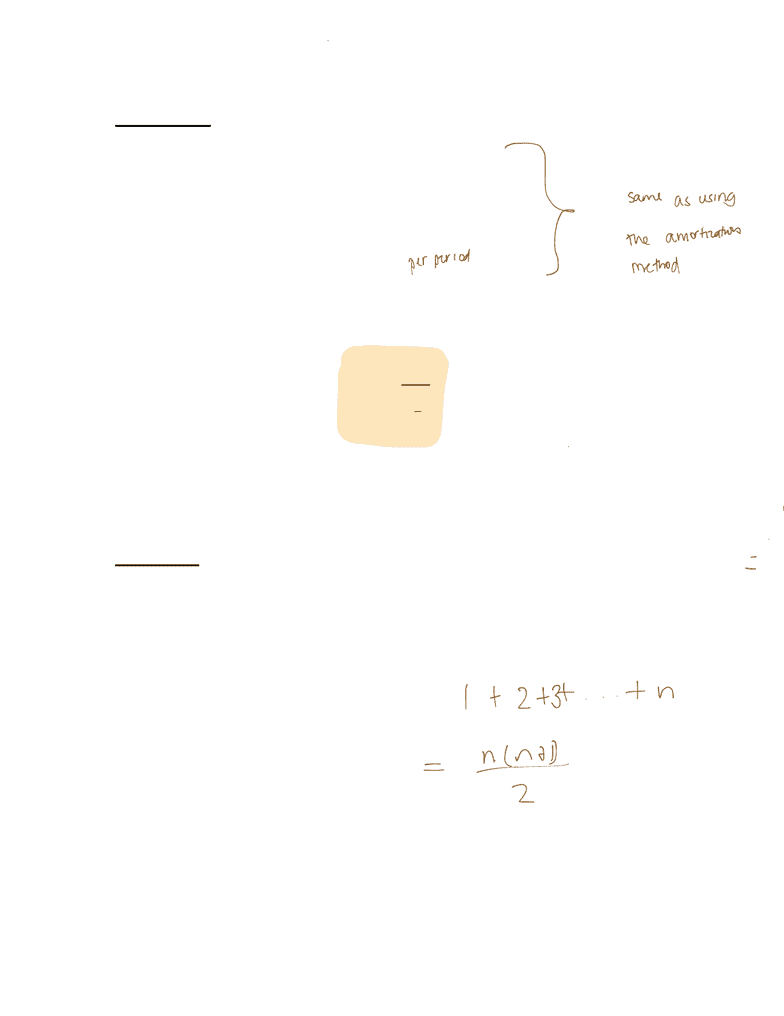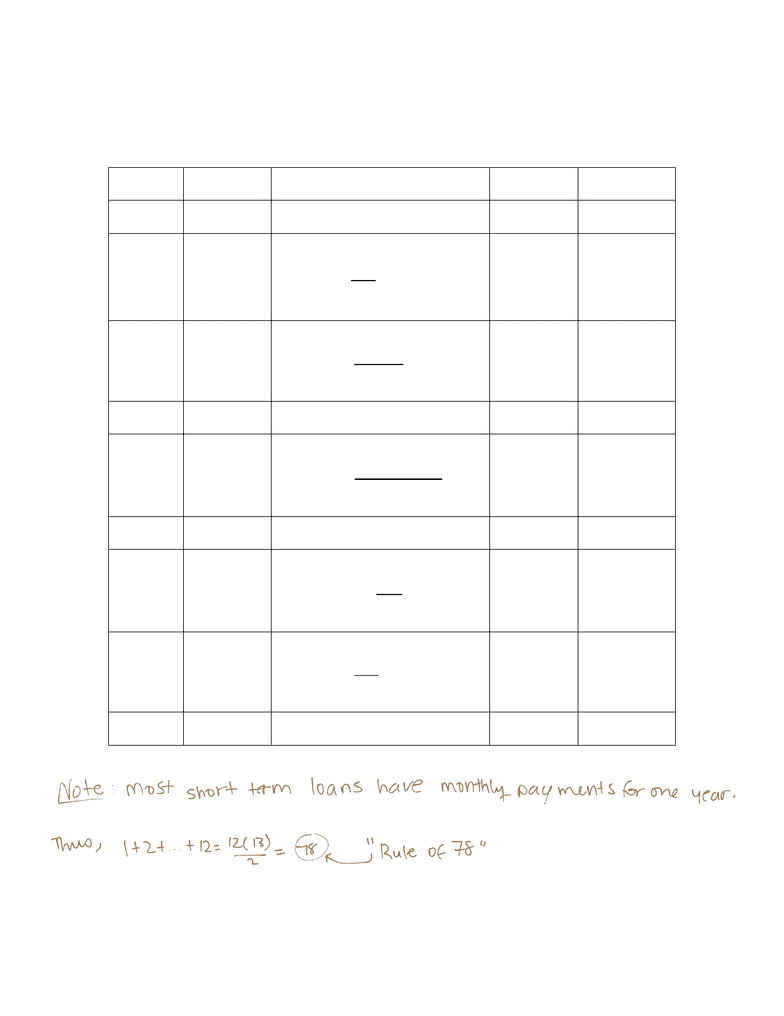Textbook Notes (270,000)
CA (160,000)
Western (10,000)
AS (20)
AS 2053 (10)
Chapter 5

Actuarial Science 2053 Chapter Notes - Chapter 5: Refinancing, Sinking Fund, 18 Months

Department
Actuarial Science
Course Code
AS 2053
Professor
Steven Kopp
Chapter
5

This preview shows pages 1-3. to view the full 22 pages of the document.Refinancing A Loan – The Sum of Digits
Method (section 5.4)
There are still a few Canadian lending
institutions that use the sum-of-digits
method (also called theRule of 78) when
determining the outstanding balance on a
loan
this method is an approximation to the
amortization method
the main difference is that we determine
the interest portion of each payment by
taking a defined proportion of the total
interest paid on the loan

Only pages 1-3 are available for preview. Some parts have been intentionally blurred.Method
Original Loan = A
Term of loan = n - periods
Interest rate on loan = i
Thus,
Periodic payment, R =
in
a
A
|
Define
1. I = total interest paid on loan = nR A
2. sum of digits 1 to n, sn =

Only pages 1-3 are available for preview. Some parts have been intentionally blurred.The interest paid column looks like:
k R I
k
P
k
B
k
0 -- -- -- A
1 R
I
s
n
I
n
=
1
2 R
I
s
n
I
n
=1
2
: : :
k R
I
s
kn
I
n
k
+
=1
: : :
n 1
R
I
s
I
n
n
=
2
1
n R
I
s
I
n
n
=1
0
total
nR I = nR A A